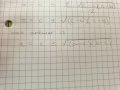# Why would completing the square give a different answer to the quadratic formula?

#### Mojo Pin__

Joined Apr 13, 2019
83
In this example from Engineering Mathematics (Glyn James) page 25/26 we are asked to use the quadratic formula to solve:

(x^2) - 2cx+c(a+b) - ab =0

My solution is:and the book solution is:I don't understand why I would get a different answer, it looks like if you complete the square you get the book answer but if you use the quadratic formula you get my answer, can someone help me understand this please?

Thanks,

Keith